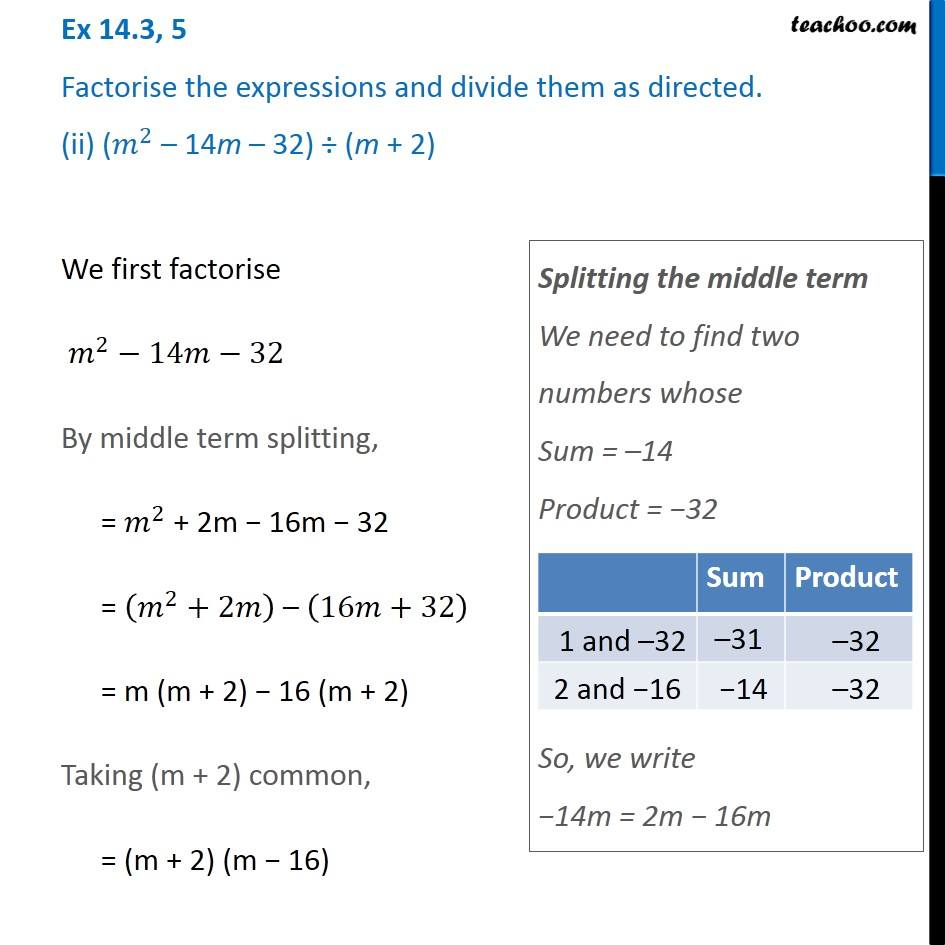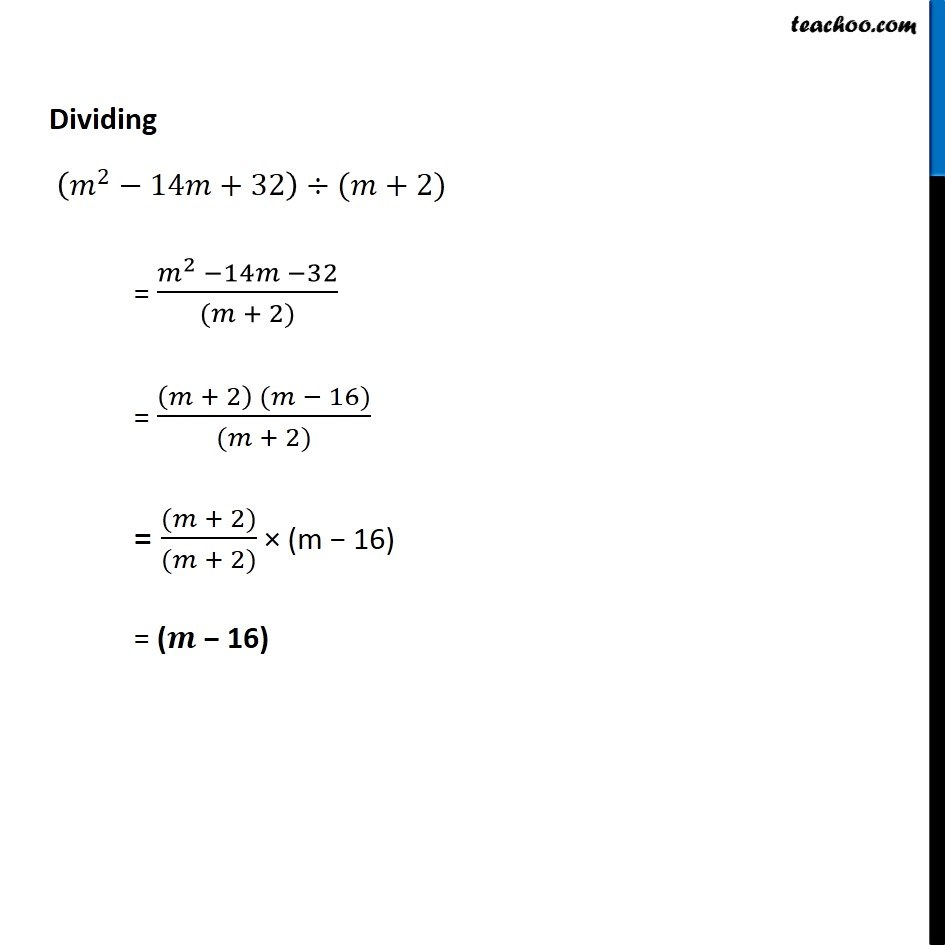Dividing two polynomials

Chapter 14 Class 8 Factorisation
Concept wiseIntroducing your new favourite teacher - Teachoo Black, at only ₹83 per month

### Transcript

Ex 14.3, 5 Factorise the expressions and divide them as directed. (ii) (𝑚^2 – 14m – 32) ÷ (m + 2) We first factorise 𝑚^2−14𝑚−32 By middle term splitting, = 𝑚^2 + 2m − 16m − 32 = (𝑚^2+2𝑚) – (16𝑚+32) = m (m + 2) − 16 (m + 2) Taking (m + 2) common, = (m + 2) (m − 16) Splitting the middle term We need to find two numbers whose Sum = –14 Product = −32 So, we write −14m = 2m − 16m Dividing (𝑚^2−14𝑚+32)÷(𝑚+2) = (𝑚^2 −14𝑚 −32)/((𝑚 + 2)) = ((𝑚 + 2) (𝑚 − 16))/((𝑚 + 2)) = ((𝑚 + 2))/((𝑚 + 2)) × (m − 16) = (𝒎 − 16)